C#图解教程 第十二章 数组

## 数组

### 数组

``````数组名  索引
↓     ↓
MyArray
``````
##### 定义

• 元素 数组的独立数据项称为元素。数组的所有元素必须是同类型的，或继承自相同的类型
• 秩/维度 数组可以有任何为正数的维度数。数组的维度数称作秩（rank）
• 维度长度 数组的每个维度都有一个长度，就是这个方向的位置个数
• 数组长度 数组的所有维度中的元素的总和称为数组的长度
##### 重要细节

• 数组一旦创建，大小就固定了。C#不支持动态数组
• 数组索引号从0开始。如果维度长度是n，索引号范围就是0到n-1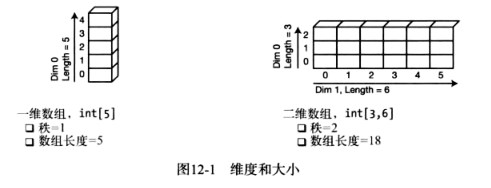### 数组的类型

C#提供两种类型的数组

• 一维数组可以认为是单行元素或元素向量
• 多维数组是由主向量中的位置组成的。每个位置本身又是一个数组，称为子数组（subarray）。子数组向量中的位置本身又是一个子数组

• 矩形数组
• 某个维度的所有子数组具有相同长度的多维数组
• 不管有多少个维度，总是使用一组方括号 `int x=myArray2[4,6,1]`
• 交错数组
• 每个子数组都是独立数组的多维度数组
• 可以有不同长度的子数组
• 为数组的每个维度使用一对方括号 `jagArray1`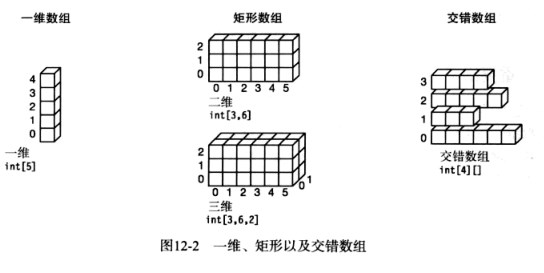### 数组是对象

• Rank 返回数组维度数
• Length 返回数组长度（数组中所有元素的个数）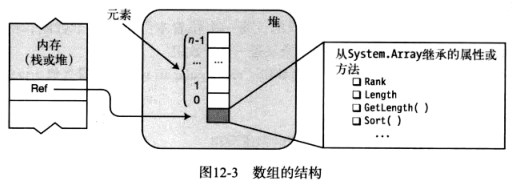• 如果存储的元素都是值类型，数组被称作值类型数组
• 如果存储的元素都是引用类型，数组被称作引用类型数组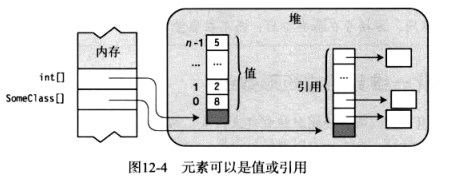### 一维数组和矩形数组

• 可以使用任意多的秩说明符

• 不能在数组类型区域中放数组维度长度。秩是数组类型的一部分，而纬度长度不是类型的一部分

• 数组声明后，维度数就固定了。然而，纬度长度直到数组实例化时才确定

秩说明符 ↓ int[,,] firstArray; //三维整型数组 int[,] arr1; //二维整型数组 long[,] arr3; //三维long数组 long[3,2,6] SecondArray;//编译错误 ↑ 声明时不允许设置维度长度

### 实例化一维数组或矩形数组

``````int[] arr2=new int;//包含4个int的一维数组
MyClass[] maArr=new MyClass;//包含4个MyClass引用的一维数组
int[,,] arr3=new int[3,6,2];//三维数组，数组长度是3*6*2=36
``````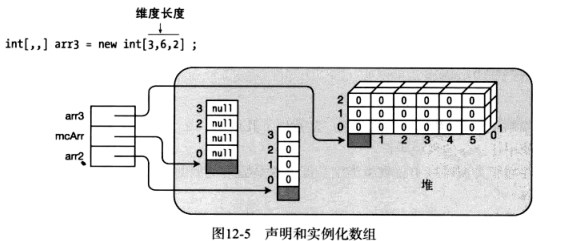### 访问数组元素

• 每个维度的索引从0开始

• 方括号内的索引在数组名称之后

int[] intArr1=new int; intArr1=10; int var1=intArr1; int[,] intArr2=new int[5,10]; intArr2[2,3]=7; int var2=intArr2[2,3];

int[] myIntArray; myIntArray=new int; for(int i=0;i<4;i++) { myIntArray[i]-i*10; } for(int i=0;i<4;i++) { Console.WriteLine("Value of element {0} = {1}",i,myIntArray[i]); }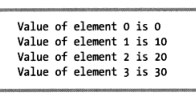### 初始化数组

``````int[] intArr=new int;
``````##### 显式初始化一维数组
• 初始值必须以逗号分隔，并封闭在一组大括号内

• 不必输入数组长度，因为编译器可以通过初始化值的个数来推断长度

• 注意，在数组创建表达式和初始化列表中间没有分隔符。即，没有等号或其他连接运算符

int[] intArr=new int[]{10,20,30,40};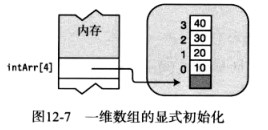##### 显式初始化矩形数组

• 每个初始值向量必须封闭在大括号内

• 每个维度也必须嵌套并封闭在大括号内

• 除了初始值，每个维度的初始化列表和组成部分也必须使用逗号分隔

int[,] intArray2=new int[,]{{10,1},{2,10},{11,9}};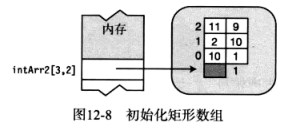##### 快捷语法

``````int[] arr1=new int{10,20,30};
int[] arr1=          {10,20,30};
int[,] arr=new int[2,3]{{0,1,2},{10,11,12}};
int[,] arr=            {{0,1,2},{10,11,12}};
``````
##### 隐式类型数组

• 当初始化数组时，我们可以让编译器根据初始化语句的类型来推断数组类型。只要所有初始化语句能隐式转换为单个类型，就可以这么做
• 和隐式类型的局部变量一样，使用var关键字

``````int[] intArr1=new int[]{10,20,30,40};
var   intArr2=new    []{10,20,30,40};
int[,] intArr3=new int[,]{{10,1},{2,10},{11,9}};
var    intArr4=new    [,]{{10,1},{2,10},{11,9}};
string[] sArr1=new string[]{"life","liberty","pursuit of happiness"};
var      sArr2=new       []{"life","liberty","pursuit of happiness"};
``````
##### 综合内容

``````var arr=new int[,]{{0,1,2},{10,11,12}};
for(int i=0;i<2;i++)
{
for(int j=0;j<3;j++)
{
Console.WriteLine("Element [{0},{1}] is {2}",i,j,arr[i,j]);
}
}
``````### 交错数组

• 第一个维度长度是3

• 声明可以读作“jagArr是3个int数组的数组”

• 图中有4个数组对象-其中一个针对顶层数组，另外3个针对子数组

int[][] jagArr=new int[];##### 声明交错数组

``````int[][] SomeArr;//秩等于2
int [][][] OhterArr;//秩等于3
``````
##### 快捷实例化

``````int[][] jagArr=new int[];//3个子数组
``````

``````int[][] jagArr=new int;//不允许，编译错误
``````
##### 实例化交错数组

1. 首先，实例化顶层数组
2. 其次，分别实例化每个子数组，把新建数组的引用赋给它们所属数组的合适元素

``````int[][] Arr=new int[];
Arr=new int[]{10,20,30};
Arr=new int[]{40,50,60,70};
Arr=new int[]{80,90,100,110,120};
``````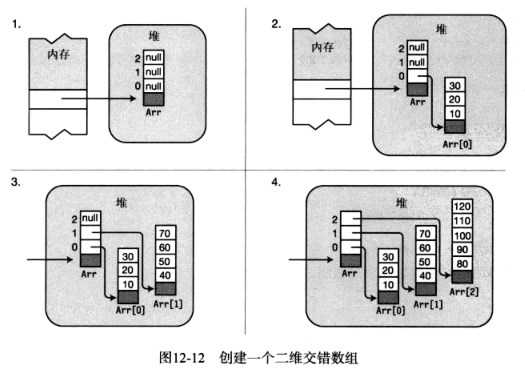### 比较矩形数组和交错数组

• 两个数组都保存9个整数，但是它们结构很不相同
• 矩形数组只有1个数组对象，而交错数组有4个数组对象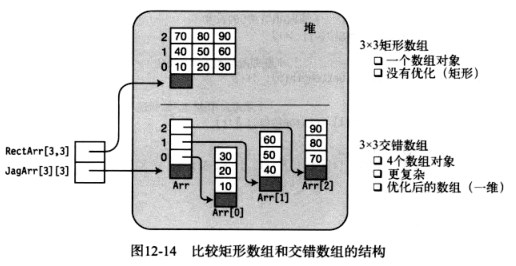### foreach语句

foreach语句允许我们连续访问数组中的每个元素。

• 迭代变量是临时的，并且和数组中元素的类型相同。foreach语句使用迭代变量来相继表示数组中的每个元素

• foreach语句的语法如下

• Type是数组中元素的类型。我们可以显式提供它的类型，或者使用var
• Identifier是迭代变量名
• ArrayName是数组名
• Statement是要为数组中每个元素执行一次的单条语句或语句块

foreach(Type Identifier in ArrayName)//显式 { Statement } foreach(var Identifier in ArrayName)//隐式 { Statement }

foreach如下方式工作：

• 从数组第一个元素开始并把它赋值给迭代变量
• 执行语句主体。在主体中，我们可以把迭代变量作为数组元素的只读别名
• 主体执行后，foreach语句选择数组中的下一个元素并重复处理

``````int[] arr1={10,11,12,13};
foreach(int item in arr1)
{
Console.WriteLine("Item Value: {0}",item);
}
``````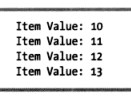##### 迭代变量是只读的

``````int[] arr1={10,11,12};
foreach(int item in arr1)
{
item++;
}
``````

``````class MyClass
{
public int MyField=0;
}
class Program
{
static void Main()
{
MyClass[] mcArray=new MyClass;
for(int i=0;i<4;i++)
{
mcArray[i]=new MyClass();
mcArray[i].MyField=i;
}
foreach(var item in mcArray)
{
item.MyField+=10;
}
foreach(var item in mcArray)
{
Console.WriteLine("{0}",item.MyField);
}
}
}
``````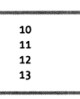##### foreach语句和多维数组

``````class Program
{
static void Main()
{
int total=0;
int[,] arr1={{10,11},{12,13}};
foreach(var element in arr1)
{
total+=element;
Console.WriteLine("Element:{0},Current Total:{1}",element,total);
}
}
}
``````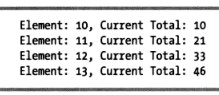``````class Program
{
static void Main()
{
int total=0;
int[][] arr1=new int[];
arr1=new int[]{10,11};
arr1=new int[]{12,13,14};
foreach(int[] array in arr1)
{
Console.WriteLine("Starting new array");
foreach(int item in array)
{
total+=element;
Console.WriteLine("Item:{0},Current Total:{1}",item,total);
}
}
}
}
``````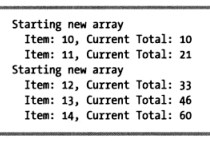### 数组协变

• 数组是引用类型数组
• 在赋值的对象类型和数组基类之间有隐式转换或显式转换

``````class A{...}
class B:A{...}
class Program
{
static void Main()
{
A[] AArray1=new A;
A[] AArray2=new A;
AArray1=new A();AArray1=new A();AArray1=new A();
AArray2=new B();AArray2=new B();AArray2=new B();
}
}
``````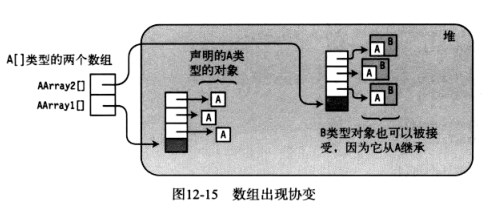### 数组继承的有用成员

C#数组从System.Array类继承。它们从基类继承了很多有用的属性和方法。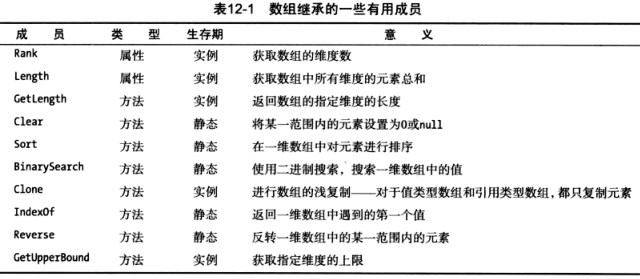``````public static void PrintArray(int[] a)
{
foreach(var x in a)
{
Console.WriteLine("{0}",x);
}
Console.WriteLine("");
}
static void Main()
{
int[] arr=new int[]{15,20,5,25,10};
PrintArray(arr);
Array.Sort(arr);
PrintArray(arr);
Array.Reverse(arr);
PrintArray(arr);
Console.WriteLine();
Console.WriteLine("Rank = {0},Length = {1}",arr.Rank,arr.Length);
Console.WriteLine("GetLength(0)      = {0}",arr.GetLength(0));
Console.WriteLine("GetType()         = {0}",arr.GetType());
}
``````##### Clone方法

Clone方法为数组进行浅复制。即，它只创建了数组本身的克隆。如果是引用类型数组，它不会复制元素引用的对象。对于值类型数组和引用类型数组，效果不同。

• 克隆值类型数组会产生两个独立数组
• 克隆引用类型数组会产生指向相同对象的两个数组

Clone方法返回object类型的引用，它必须被强制转换成数组类型。

``````int[] intArr1={1,2,3};
int[] intArr2=(int[])intArr1.Clone();
``````

``````class Program
{
static void Main()
{
int[] intArr1={1,2,3};
int[] intArr2=(int[])intArr1.Clone();
intArr2=100;
intArr2=200;
intArr2=300;
}
}
````````````class A
{
public int Value=5;
}
class Program
{
static void Main()
{
A[] AArray1=new A{new A(),new A(),new A()};
A[] AArray2=(A[])AArray1.Clone();
AArray2.Value=100;
AArray2.Value=200;
AArray2.Value=300;
}
}
``````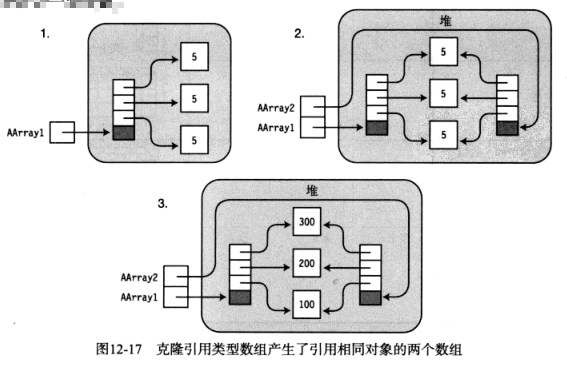### 比较数组类型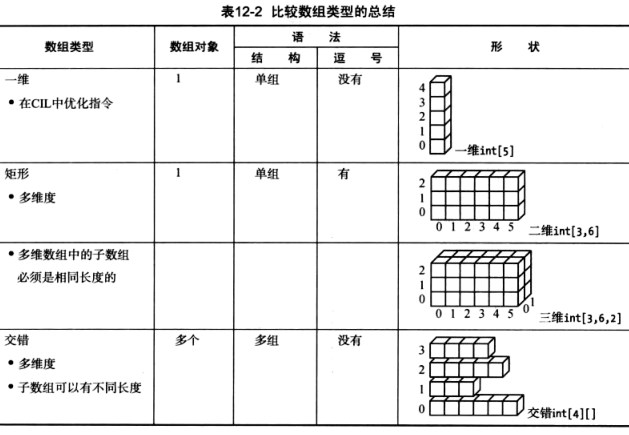blmius
2年前
MySQL:[Err] 1292 - Incorrect datetime value: ‘0000-00-00 00:00:00‘ for column ‘CREATE_TIME‘ at row 1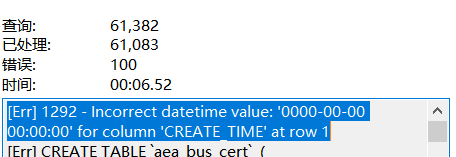2年前
js 数组 转为树形结构Wesley13
1年前
java将前端的json数组字符串转换为列表helloworld_34035044
1年前

​在我们用皕杰报表工具设计填报报表时，如何在新增行里自动增加id呢？能新增整数排序id吗？目前可以在新增行里自动增加id，但只能用uuid函数增加UUID编码，不能新增整数排序id。uuid函数说明：获取一个UUID，可以在填报表中用来创建数据ID语法：uuid()或uuid(sep)参数说明：sep布尔值，生成的uuid中是否包含分隔符''，缺省为Wesley13
1年前
Java开发者容易犯的十个错误
!(https://oscimg.oschina.net/oscnet/c9f00cc918684fbe8a865119d104090b.gif)Top1.数组转换为数组列表将数组转换为数组列表，开发者经常会这样做：\java\List<StringlistArrays.asList(arr);ArrStella981
1年前
JS 对象数组Array 根据对象object key的值排序sort,很风骚哦Wesley13
1年前
JAVA 基础3Wesley13
1年前
ES6 新增的数组的方法Wesley13
1年前
C语言自学《五》京东云开发者
10小时前

UUID​UUID（通用唯一识别码）是由32个十六进制数组成的无序字符串，通过一定的算法计算出来。为了保证其唯一性，UUID规范定义了包括网卡MAC地址、时间戳、名字空间（Namespace）、随机或伪随机数、时序等元素，以及从这些元素生成UUID的算法。Wesley13
Lv1

1.2w

18

1.7k